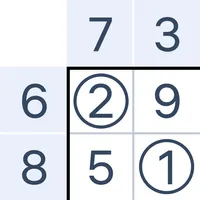# Number Sums - Numbers Game

Easybrain
Free
4.7 out of 5

## About Number Sums - Numbers Game

Number Sums is a challenging number puzzle to train your mental arithmetic. The goal is to make the sum of numbers in each row and column be equal to the clues on the side of the board. Test your math skills and logic with Number Sums!

Each row and column has its own solution, but the goal of this math game is to make them all work at the same time. You have to circle correct numbers and erase numbers you don't need. Remember, the sums of numbers in rows and columns should be equal to the numbers on the side of the board. Each level of this numbers game has only one solution, try to find it to solve challenging mind puzzles!

Mental arithmetic is an invaluable math skill for your everyday life. Inside Number Sums you'll find math puzzles of various difficulty. The mechanics of these math riddles sounds simple but requires a lot of thinking. Playing sum number games and solving math problems can improve your arithmetic skills. If you are interested in number puzzles or math games for adults, and in training your addition skills, play this engaging number game for hours of fun!

How to play Number Sums:
- Circle the correct numbers that add up to the values on the side of rows and columns.
- Use the toggle to switch between circling and erasing modes. It will help you to mark the correct numbers and the extra numbers that you don't need.
- Each level of these math puzzles has only one possible solution, so make sure that rows and columns work together at the same time.
- Train your math skills with this free number puzzle with various levels of difficulty. Open different types of board from 3x3 to 10x10.